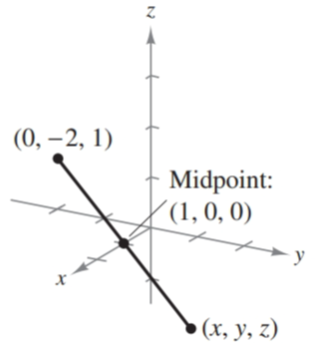Chapter 7.1, Problem 20E### Calculus: An Applied Approach (Min...

10th Edition
Ron Larson
ISBN: 9781305860919

#### Solutions

Chapter
Section### Calculus: An Applied Approach (Min...

10th Edition
Ron Larson
ISBN: 9781305860919
Textbook Problem
1 views

# Using the Midpoint Formula in Space In Exercises 19-22, find (x, y, z).To determine

To calculate: The endpoint coordinate (x,y,z) from the provided figure.Explanation

Given Information:

The provided figure is,

Formula used:

Midpoint formula in space, the midpoint of the line segment joining the points (x1,y1,z1) and (x2,y2,z2).

Midpoint=(x1+x22,y1+y22,z1+z22)

Calculation:

From the provided figure, the midpoint of the line segment is (1,0,0). The endpoint of the line segment is (0,2,1) and (x,y,z)

By the use of midpoint formula in space,

Midpoint=(x1+x22,y1+y22,z1+z22)(1,0,0)=(

### Still sussing out bartleby?

Check out a sample textbook solution.

See a sample solution

#### The Solution to Your Study Problems

Bartleby provides explanations to thousands of textbook problems written by our experts, many with advanced degrees!

Get Started

#### Completely factor each of the following.

Mathematical Applications for the Management, Life, and Social Sciences

#### Find the limit. limr9r(r9)4

Single Variable Calculus: Early Transcendentals

#### The radius of convergence of is: 1 3 ∞

Study Guide for Stewart's Multivariable Calculus, 8th

#### Describe the general characteristics of the survey research design.

Research Methods for the Behavioral Sciences (MindTap Course List)